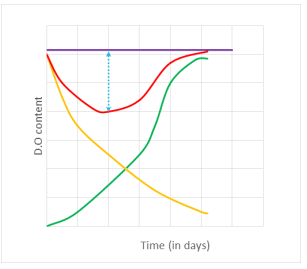# Test: Oxygen Sag Curve

## 5 Questions MCQ Test Environmental Engineering | Test: Oxygen Sag Curve

Description
Attempt Test: Oxygen Sag Curve | 5 questions in 25 minutes | Mock test for Chemical Engineering preparation | Free important questions MCQ to study Environmental Engineering for Chemical Engineering Exam | Download free PDF with solutions
QUESTION: 1

### The yellow curve representsSolution:

Explanation: The yellow curve represents the deoxygenation curve, which depicts the depletion of dissolved oxygen in the absence of aeration.

QUESTION: 2

### Which of the following is represented by the green curve?Solution:

Explanation: The green curve represents the reoxygenation curve, which depicts the supply of oxygen from the atmosphere to counterbalance the oxygen deficit.

QUESTION: 3

### The maximum deficiency of dissolved oxygen is represented bySolution:

Explanation: The maximum deficiency of dissolved oxygen is represented by the dotted blue line. It is finding with respect to the equilibrium concentration of oxygen.

QUESTION: 4

Which of the following represents the oxygen sag curve?Solution:

Explanation: The oxygen sag curve is represented by the red curve. It is also called as an oxygen deficit curve.

QUESTION: 5

The oxygen deficit is maximum whenSolution:

Explanation: The rate of oxygen deficit is the sum of the rate of reaeration and the rate of deoxygenation and it is maximum when the rate of reaeration equals the rate of deoxygenation.Use Code STAYHOME200 and get INR 200 additional OFF Use Coupon Code